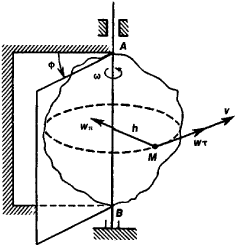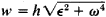# Rotational Motion of Rigid Bodies

The following article is from The Great Soviet Encyclopedia (1979). It might be outdated or ideologically biased.

## Rotational Motion of Rigid Bodies

(1) Rotation about an axis—the motion of a rigid body in which any two of its points A and B always remain motionless (see Figure 1).Figure 1. Rotation of a rigid body about an axis

The straight line AB that passes through these points is called the axis of rotation. During rotation all points of the body describe circles in planes perpendicular to the rotation axis and with centers lying along this axis. A rotating body has one degree of freedom, and its position is determined by the angle Φ between the fixed half-plane passing through the axis of rotation and the half-plane rigidly attached to the body and rotating with it. The basic kinematic characteristics of rotational motion of a body are its angular velocity co and its angular acceleration e. For any point of the body at distance h from the axis, the linear velocity v = hw, the tangential acceleration WT = he, the normal acceleration wnhw)2, and the total acceleration w =The basic dynamic characteristics of a rotating body are the angular momentum relative to the axis of rotation Kz = Izw) and the kinetic energy T = l/2lzw)2, where Iz is the moment of inertia of the body about the axis of rotation. The law of rotation is defined from the basic equation Ize = Mz where Mz is the torque moment.

(2) Rotation around a point, or spherical motion, is the motion of a rigid body during which one of its points O remains fixed, while all the other points move along the surface of spheres with their center at point O. During such rotation of a rigid body, any elementary displacement of the body is an elementary rotation around some axis passing through point O and called the instantaneous axis of rotation. This axis, unlike a fixed axis, is constantly changing its direction with time. As a result, the rotational motion of a rigid body consists of a series of elementary rotations about instantaneous axes that are constantly changing direction. An example of such rotation is the movement of a gyroscope.

S. M. TARG